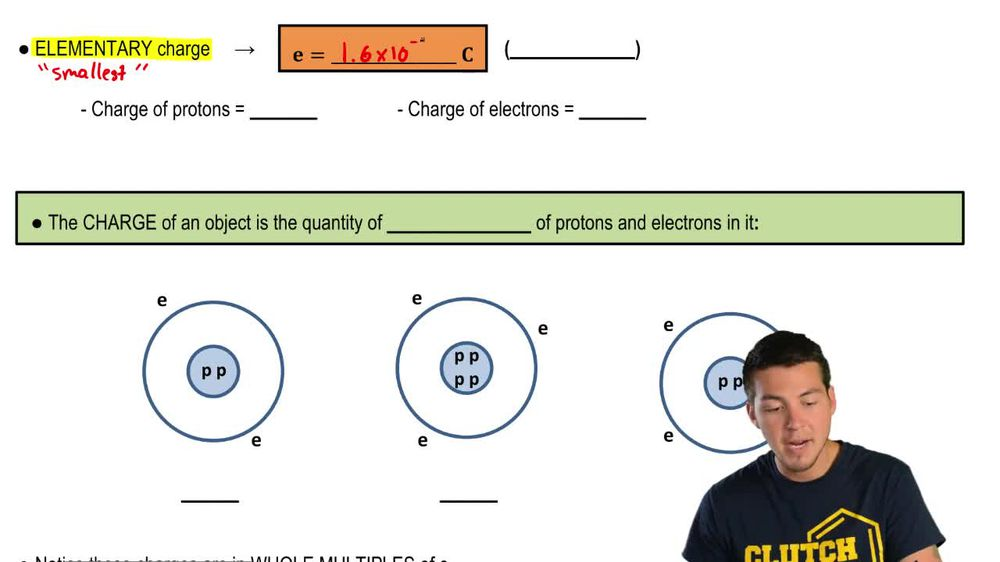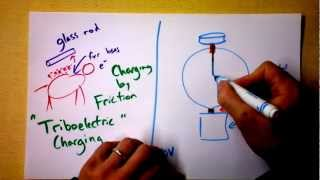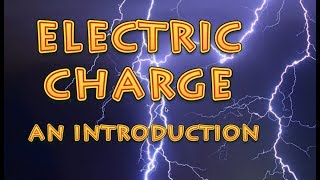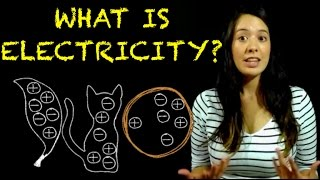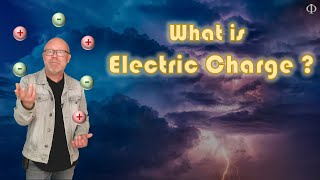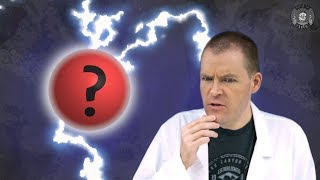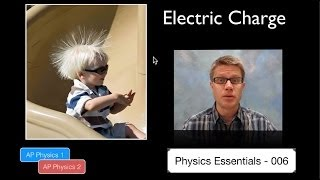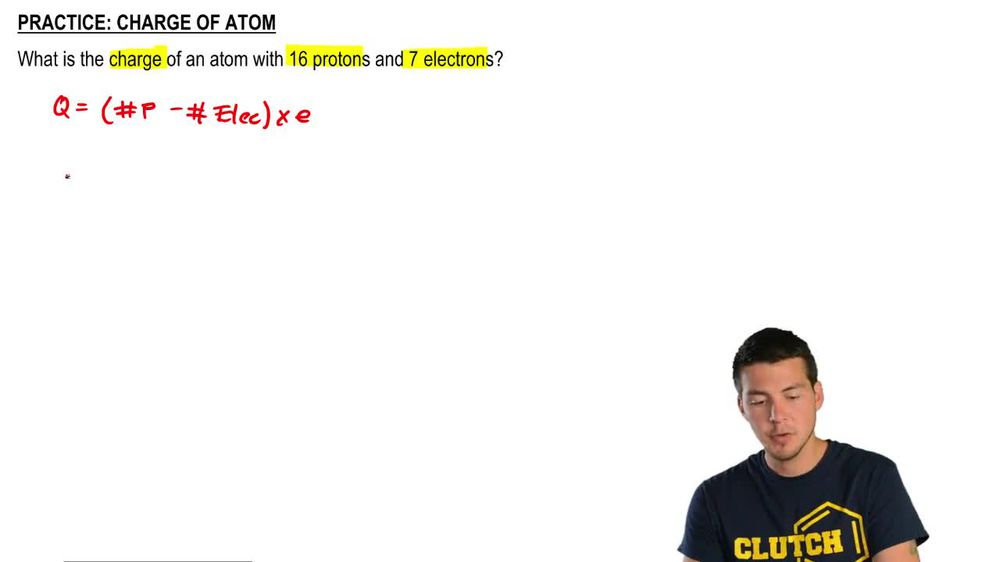Start typing, then use the up and down arrows to select an option from the list.
1. 24. Electric Force & Field; Gauss' Law2. Electric Charge# Charge of Atom

by Patrick Ford
238 views
5
5
Hey, guys, hopefully able to figure this one out on your own? So in this problem were asked how What is the total charge of an atom? We're told the amount of protons and the amount of electrons. So let's go ahead and use our charge equation. So we know that charge is equal to we've got the number of protons minus the number of electrons, and we're gonna multiply that by the elementary charge. So the total charge should be We've got 16 protons, minus seven electrons, and that's a that's a seven right there. And we're gonna multiply by the elementary charge, which is 1.6 times 10 to the minus 19. You're gonna need to remember that number. So go ahead and commit it to memory right now. So we've got two. Q is equal to you. Go ahead and work all this out. It should be nine times 1.6 times 10 to the minus 19 and that's a total charge of 1.44 times 10 to the minus 18. Cool. OEMs. So that's the total amount of charge, and what I want you to do is notice that it's also positive and that makes sense because we have more protons, their arm or protons than electrons. So we should end up with a positive number. Let me know if you guys have any questions.